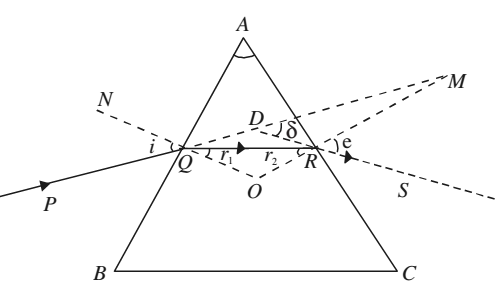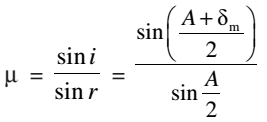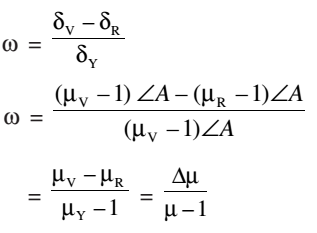# Angle of Deviation

Let a monochromatic beam of light PQ is incident on the face AB of the principal section of the prism ABC. On refraction, it goes along QR inside the prism and emerges along RS from face AC.

∠NQP = ∠i (angle of incidence)

∠MRS = ∠e (angle of emergence)

∠RQO = ∠r1 (angle of refraction at the face AB)

∠QRO = ∠r2 (angle of refraction at the face AC)

The angle between the emergent ray RS and the incident ray PQ at D is known as the angle of deviation (δ).Since ∠MDR = ∠δ, As it is the external angle of the triangle QDR,

∠δ = ∠DQR + ∠DRQ

= (∠i – ∠r1) + (∠e – ∠r2)

∠δ = (∠i + ∠e) – (∠r1 + ∠r2)

The sum of the internal angles of a quadrilateral is equal to 360°. In the quadrilateral AQOR, ∠AQO = ∠ARO = 90°, since NQ and MR are normal on faces AB and AC, respectively. Therefore

∠QAR + ∠QOR= 180°

∠A + ∠QOR = 180°

But in ∆QOR

∠OQR + ∠QRO + ∠QOR= 180°

∠r1 + ∠r2 + ∠QOR = 180°

On comparing two equations,

∠r1 + ∠r2 = ∠A

∠δ = (∠i + ∠e) – ∠A

∠i + ∠e = ∠A + ∠δ

### Angle of Minimum Deviation

If you vary the angle of incidence i, the angle of deviation δ also changes. It becomes minimum for a certain value of i and again starts increasing as i increases further. The minimum value of the angle of deviation is called angle of minimum deviation (δm). It depends on the material of the prism and the wavelength of light used.

The light beam inside the prism, under the condition of minimum deviation, passes symmetrically through the prism and is parallel to its base. The refractive index of the material of the prism is given by### Angular Dispersion and Dispersive Power

The difference between the angles of deviation for any two wavelengths (colors) is known as the angular dispersion for those wavelengths. The angular dispersion between the red and violet wavelengths is δV – δR. In the visible part of the spectrum, the wavelength of the yellow color is nearly the average wavelength of the spectrum. The deviation for this color δY is taken as the average of all deviations.

The ratio of the angular dispersion to the mean deviation is taken as the dispersive power (ω) of the material of the prism.